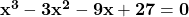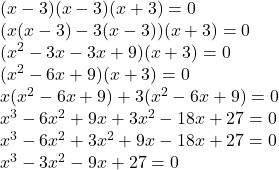what is a cubic polynomial function with zeros 3,3 and -3? show your work.​

Question

what is a cubic polynomial function with zeros 3,3 and -3? show your work.​

in progress 0
4 months 2021-09-03T06:02:40+00:00 1 Answers 3 views 0

Cubic polynomial function with zeros 3,3 and -3 isStep-by-step explanation:

We need to find a cubic polynomial function with zeros 3,3 and -3.

If zeros of polynomial are: 3,3,and -3

we can write:

x=3, x=3, x=-3

Or

We can write:

x-3=0, x-3=0, x+3=0

Now, we can write them as:

(x-3)(x-3)(x+3)=0

Multiplying the terms, we can find the polynomial:So, cubic polynomial function with zeros 3,3 and -3 is